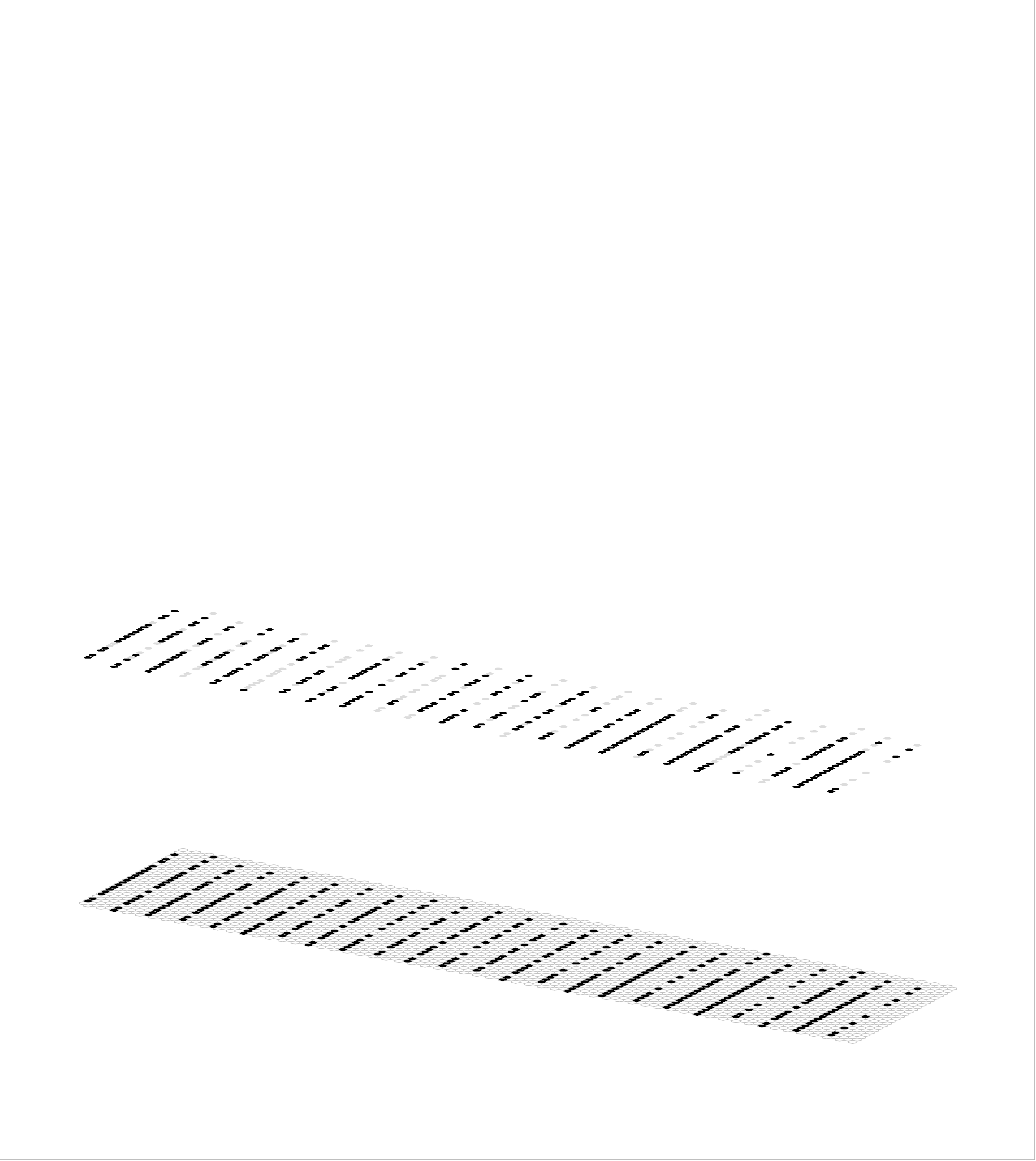# Montage of dense HOF grid depictions of Weizmann Videos

This montage shows 5 instances of each the wave2, bend and walk classes from Weizmann data set processed as an 8x8 grid of HOF vectors, where each HOF vector is a 3x15 binary vector, which is parsed as a 3x3 grid of 5-bit vectors, where the 5 bits are the binary-thresholded bins of the 5-bin histograms, where the bins correspond to no motion, NE, SE, SW, and NW. The 15 snippets have synchronized starts they have different lengths.  There are about 4 overall cycles and the snippets resync at the beginning of each cycle.

You can use ctrl+<left/right-arrow> to step back/forward through frames.

These HOF snippets are of course much harder to understand than the edge snippets we've been using as inputs heretofore.

Below shows a 4-level Sparsey processing the Daria-Bend snippet (upper middle panel above), showing which of the active input features (pixels) actually get represented (black) at each higher level and thus figure in the classification process. Gray pixels are not represented at the level in wwhich they are shown. Note that this network has 12x30, 4x12, and 2x3 mac grids at levels V1, V2, and V3.  In general, we would rather have it be the case that all pixels are represented at all levels (remain black at all levels) because only in that case, is the model's final output [which is the union of sparse distributed codes active in the top level (which consists of 6 macs in this case) and which is not shown here] a function of all the information present in the snippet.  To achieve this, we need to find the correct general parameter settings (within and between levels).  In the particular case shown below, large fractions of the active input features on each frame end up not influencing the codes in the top-level.Note that the question of whether or not an input feature (pixel) influences the choice of code at a given level is not the same question as whether or not that pixel (or more generally, any larger group of active pixels) constitutes a feature that is useful to the classificaiton task. So we're not talking about the issue of discarding semantically irrelevant features here (cf. compression). In other words, this dropping out of the influence of input features shown here is generally not desirable.